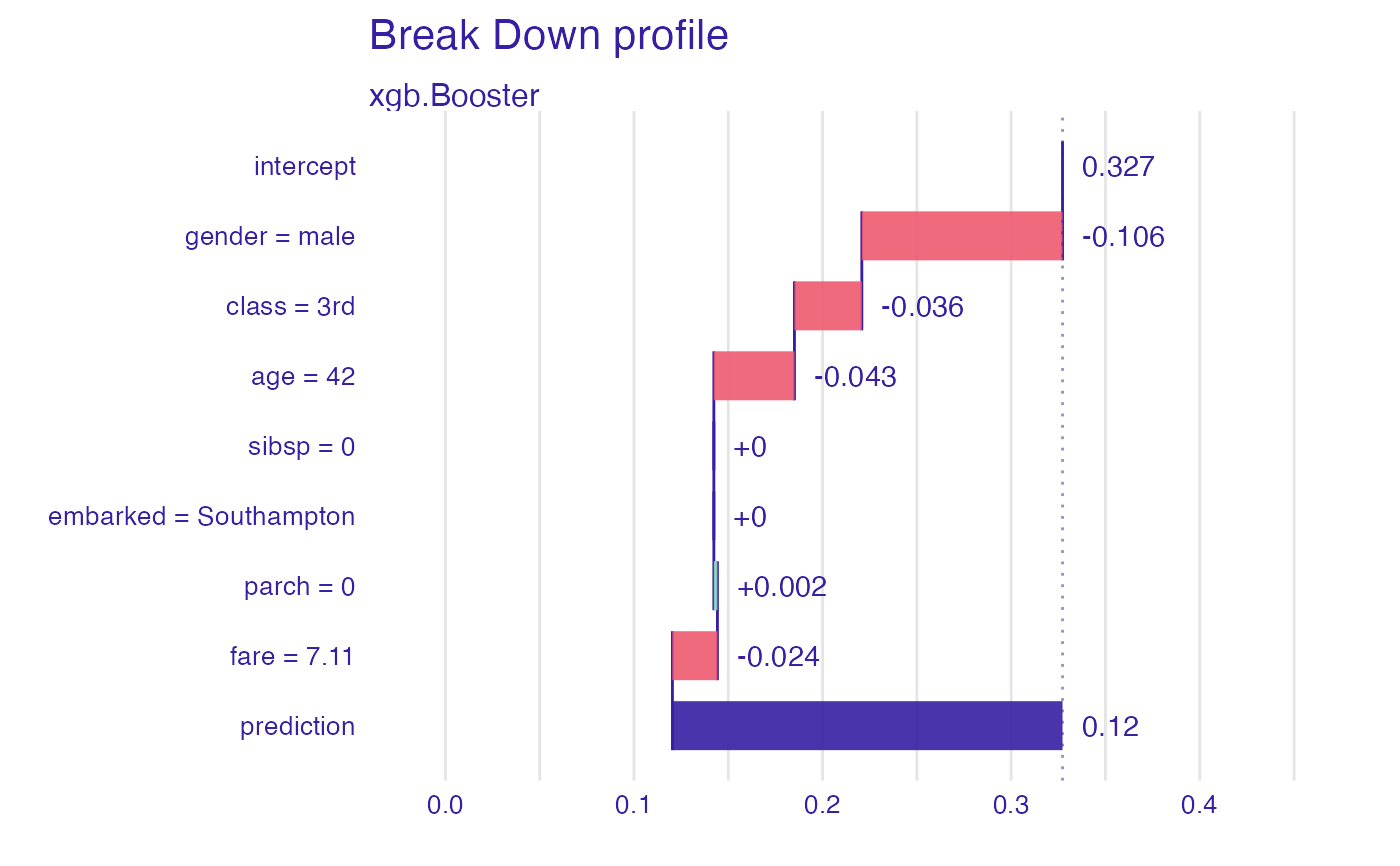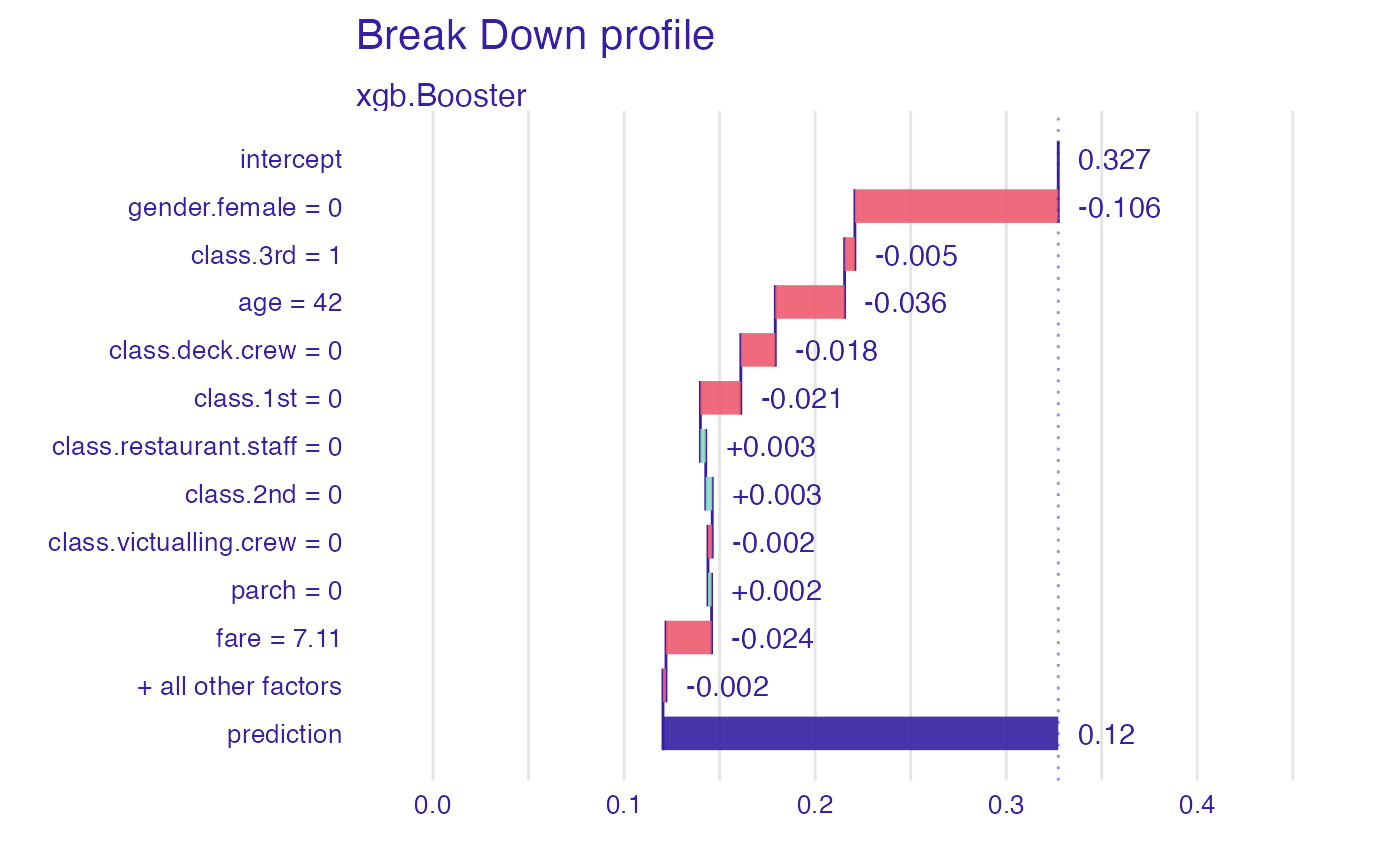DALEX is designed to work with various black-box models like tree ensembles, linear models, neural networks etc. Unfortunately R packages that create such models are very inconsistent. Different tools use different interfaces to train, validate and use models. One of those tools, we would like to make more accessible is the xgboost package.

explain_xgboost(
model,
data = NULL,
y = NULL,
weights = NULL,
predict_function = NULL,
predict_function_target_column = NULL,
residual_function = NULL,
...,
label = NULL,
verbose = TRUE,
precalculate = TRUE,
colorize = !isTRUE(getOption("knitr.in.progress")),
model_info = NULL,
type = NULL,
encode_function = NULL,
true_labels = NULL
)

## Arguments

model

object - a model to be explained

data

data.frame or matrix - data which will be used to calculate the explanations. If not provided, then it will be extracted from the model. Data should be passed without a target column (this shall be provided as the y argument). NOTE: If the target variable is present in the data, some of the functionalities may not work properly.

y

numeric vector with outputs/scores. If provided, then it shall have the same size as data

weights

numeric vector with sampling weights. By default it's NULL. If provided, then it shall have the same length as data

predict_function

function that takes two arguments: model and new data and returns a numeric vector with predictions. By default it is yhat.

predict_function_target_column

Character or numeric containing either column name or column number in the model prediction object of the class that should be considered as positive (i.e. the class that is associated with probability 1). If NULL, the second column of the output will be taken for binary classification. For a multiclass classification setting, that parameter cause switch to binary classification mode with one vs others probabilities.

residual_function

function that takes four arguments: model, data, target vector y and predict function (optionally). It should return a numeric vector with model residuals for given data. If not provided, response residuals ($$y-\hat{y}$$) are calculated. By default it is residual_function_default.

...

other parameters

label

character - the name of the model. By default it's extracted from the 'class' attribute of the model

verbose

logical. If TRUE (default) then diagnostic messages will be printed

precalculate

logical. If TRUE (default) then predicted_values and residual are calculated when explainer is created. This will happen also if verbose is TRUE. Set both verbose and precalculate to FALSE to omit calculations.

colorize

logical. If TRUE (default) then WARNINGS, ERRORS and NOTES are colorized. Will work only in the R console. Now by default it is FALSE while knitting and TRUE otherwise.

model_info

a named list (package, version, type) containing information about model. If NULL, DALEX will seek for information on it's own.

type

type of a model, either classification or regression. If not specified then type will be extracted from model_info.

encode_function

function(data, ...) that if executed with data parameters returns encoded dataframe that was used to fit model. Xgboost does not handle factors on it's own so such function is needed to acquire better explanations.

true_labels

a vector of y before encoding.

## Value

explainer object (explain) ready to work with DALEX

## Examples

library("xgboost")
#>
#> Attaching package: ‘xgboost’
#> The following object is masked from ‘package:dplyr’:
#>
#>     slice
library("DALEXtra")
library("mlr")
# 8th column is target that has to be omitted in X data
data <- as.matrix(createDummyFeatures(titanic_imputed[,-8]))
model <- xgboost(data, titanic_imputed$survived, nrounds = 10, params = list(objective = "binary:logistic"), prediction = TRUE) #> [00:35:43] WARNING: amalgamation/../src/learner.cc:627: #> Parameters: { "prediction" } might not be used. #> #> This could be a false alarm, with some parameters getting used by language bindings but #> then being mistakenly passed down to XGBoost core, or some parameter actually being used #> but getting flagged wrongly here. Please open an issue if you find any such cases. #> #> #>  train-logloss:0.574286 #>  train-logloss:0.509887 #>  train-logloss:0.472243 #>  train-logloss:0.447027 #>  train-logloss:0.428637 #>  train-logloss:0.415121 #>  train-logloss:0.405934 #>  train-logloss:0.399623 #>  train-logloss:0.394859 #>  train-logloss:0.391072 # explainer with encode functiom explainer_1 <- explain_xgboost(model, data = titanic_imputed[,-8], titanic_imputed$survived,
encode_function = function(data) {
as.matrix(createDummyFeatures(data))
})
#> Preparation of a new explainer is initiated
#>   -> model label       :  xgb.Booster  (  default  )
#>   -> data              :  2207  rows  7  cols
#>   -> target variable   :  2207  values
#>   -> predict function  :  yhat.xgb.Booster  will be used (  default  )
#>   -> predicted values  :  No value for predict function target column. (  default  )
#>   -> model_info        :  package xgboost , ver. 1.6.0.1 , task classification (  default  )
#>   -> predicted values  :  numerical, min =  0.04738454 , mean =  0.3272404 , max =  0.9705966
#>   -> residual function :  difference between y and yhat (  default  )
#>   -> residuals         :  numerical, min =  -0.8105438 , mean =  -0.005083592 , max =  0.9452911
#>   A new explainer has been created!
plot(predict_parts(explainer_1, titanic_imputed[1,-8]))# explainer without encode function
explainer_2 <- explain_xgboost(model, data = data, titanic_imputed\$survived)
#> Preparation of a new explainer is initiated
#>   -> model label       :  xgb.Booster  (  default  )
#>   -> data              :  2207  rows  17  cols
#>   -> target variable   :  2207  values
#>   -> predict function  :  yhat.xgb.Booster  will be used (  default  )
#>   -> predicted values  :  No value for predict function target column. (  default  )
#>   -> model_info        :  package xgboost , ver. 1.6.0.1 , task classification (  default  )
#>   -> predicted values  :  numerical, min =  0.04738454 , mean =  0.3272404 , max =  0.9705966
#>   -> residual function :  difference between y and yhat (  default  )
#>   -> residuals         :  numerical, min =  -0.8105438 , mean =  -0.005083592 , max =  0.9452911
#>   A new explainer has been created!
plot(predict_parts(explainer_2, data[1,,drop = FALSE]))